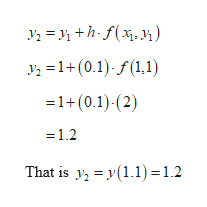# Use five iterations, y6, of Euler's method to obtain an approximation of the indicated value with h=0.1. Calculate the absolute error by comparing the approximate solution with the actual solution of the initial value problem at the indicated value.y'=2xy, y(1)=1, Y(1.5)

Question
45 views

Use five iterations, y6, of Euler's method to obtain an approximation of the indicated value with h=0.1. Calculate the absolute error by comparing the approximate solution with the actual solution of the initial value problem at the indicated value.

y'=2xy, y(1)=1, Y(1.5)

check_circle

Step 1

Consider the provided intitial value problem.

Step 2

According to euler’s method:

Step 3

Now substitute h = 0.1  x1 = 1, y1 = 1 in euler&r...help_outlineImage TranscriptioncloseУ, %3D у +h-f(x, ) У, %3D1+ (0.1)-f(1,1) =1+(0.1) (2) 1.2 That is yy1.1) 1.2 fullscreen

### Want to see the full answer?

See Solution

#### Want to see this answer and more?

Solutions are written by subject experts who are available 24/7. Questions are typically answered within 1 hour.*

See Solution
*Response times may vary by subject and question.
Tagged in

### Math